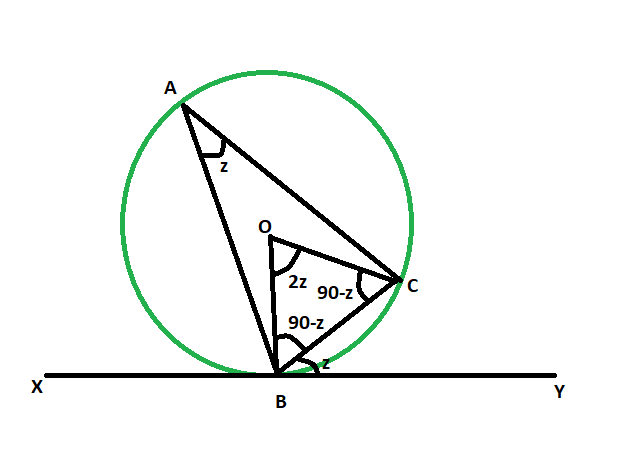Open In App

# Angle between a chord and a tangent when angle in the alternate segment is given

Given a circle whose chord and tangent meet at a particular point. The angle in the alternate segment is given. The task here is to find the angle between the chord and the tangent.
Examples:

```Input: z = 48
Output: 48 degrees

Input: z = 64
Output: 64 degrees```Approach

• Let, angle BAC is the given angle in the alternate segment.
• let, the angle between the chord and circle = angle CBY = z
• as line drawn from center on the tangent is perpendicular,
• so, angle OBC = 90-z
• as, OB = OC = radius of the circle
• so, angle OCB = 90-z
• now, in triangle OBC
angle OBC + angle OCB + angle BOC = 180
angle BOC = 180 – (90-z) – (90-z)
angle BOC = 2z
• as angle at the circumference of a circle is half the angle at the centre subtended by the same arc,
so, angle BAC = z
• hence, angle BAC = angle CBYBelow is the implementation of the above approach:

## C++

 `// C++ program to find the angle``// between a chord and a tangent``// when angle in the alternate segment is given` `#include ``using` `namespace` `std;` `void` `anglechordtang(``int` `z)``{``    ``cout << ``"The angle between tangent"``         ``<< ``" and the chord is "``         ``<< z << ``" degrees"` `<< endl;``}` `// Driver code``int` `main()``{``    ``int` `z = 48;``    ``anglechordtang(z);``    ``return` `0;``}`

## Java

 `// Java program to find the angle``// between a chord and a tangent``// when angle in the alternate segment is given``import` `java.io.*;` `class` `GFG``{` `    ``static` `void` `anglechordtang(``int` `z)``    ``{``        ``System.out.print( ``"The angle between tangent"``            ``+ ``" and the chord is "``            ``+ z + ``" degrees"``);``    ``}``    ` `    ``// Driver code``    ``public` `static` `void` `main (String[] args)``    ``{``        ``int` `z = ``48``;``        ``anglechordtang(z);``    ``}``}` `// This code is contributed by anuj_67..`

## Python3

 `# Python3 program to find the angle``# between a chord and a tangent``# when angle in the alternate segment is given``def` `anglechordtang(z):` `    ``print``(``"The angle between tangent"``,``          ``"and the chord is"``, z , ``"degrees"``);` `# Driver code``z ``=` `48``;``anglechordtang(z);` `# This code is contributed``# by Princi Singh`

## C#

 `// C# program to find the angle``// between a chord and a tangent``// when angle in the alternate segment is given``using` `System;` `class` `GFG``{` `    ``static` `void` `anglechordtang(``int` `z)``    ``{``        ``Console.WriteLine( ``"The angle between tangent"``            ``+ ``" and the chord is "``            ``+ z + ``" degrees"``);``    ``}``    ` `    ``// Driver code``    ``public` `static` `void` `Main ()``    ``{``        ``int` `z = 48;``        ``anglechordtang(z);``    ``}``}` `// This code is contributed by anuj_67..`

## Javascript

 ``

Output:

`The angle between tangent and the chord is 48 degrees`

Time Complexity: O(1)

Auxiliary Space: O(1)